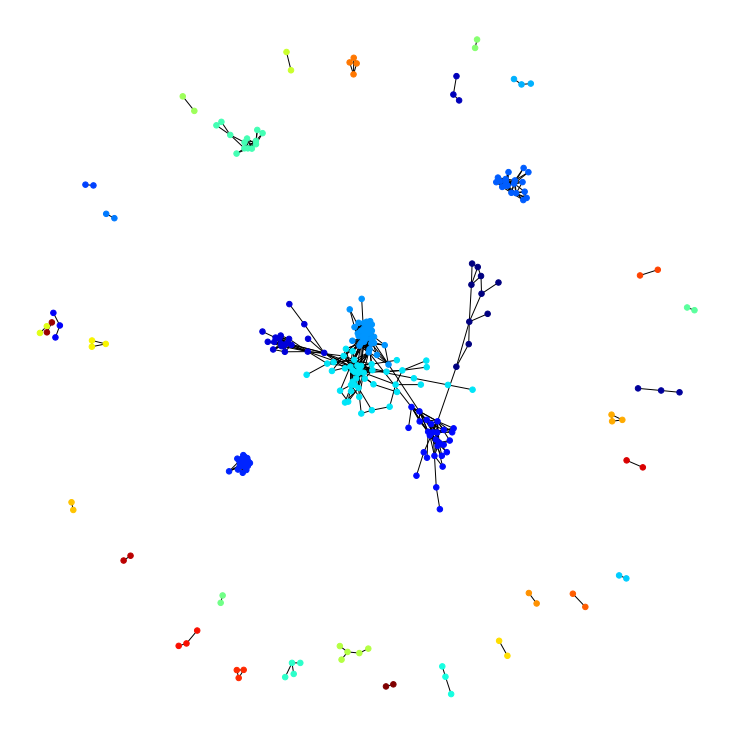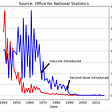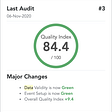# Community Detection & Network Analysis of the Stock Market in Python & R — Part 2

This is a continuation of the previous blog which showcased data scraping and cleaning. We will now move to modelling our network and form communities using clustering algorithms that will eventually be used to create our portfolio that could beat the SPY average YoY return of 8.93%.

## Part 2: Modelling

This end to end solution architecture shows how stock information will be transformed into a network that builds communities of correlated stocks by price movement over time.

The next step is to build the relationship between the stocks by calculating the correlation coefficient as shown below.

Time Series Cross — Correlation Calculation:

We will create a function to calculate the cross-correlation of stocks over different time windows.

`# Function to calculate corrdef calculate_corr(df_stock_returns, returns_window, corr_window_size, corr_method):    stocks_cross_corr_dict = {}    #Calculate mean correlation by window for plot    x_days = []    y_mean_corr = []        #     W = corr_window_size    for i in range(returns_window,len(df_stock_returns),corr_window_size):        dic_key = i        stocks_cross_corr_dict[dic_key]=df_stock_returns.iloc[i:(i+W)].corr(method='pearson')        stocks_cross_corr_dict[dic_key].fillna(0,inplace=True)        x_days.append(dic_key)        y_mean_corr.append(np.mean([abs(j) for j in stocks_cross_corr_dict[dic_key].values.flatten().tolist()]))            return stocks_cross_corr_dict, x_days,y_mean_corr`

Once the correlation function is made we can visualize it over different time windows. In the code below, we choose 21 as the time window and computed it over 5 rolling windows.

`%matplotlib inline# stocks_cross_corr_dict = {}#Time Window width#TO DO: try different windows and different algorithms#t= 21 #21 based on the paper Asset trees and asset graphs in financial markets J.-P. Onnela et all# Try window from 1 month to 6 months of trading days# 21 days is one month trading daysstart = 21end = 126step = 21;plt.figure(figsize=(20, 10))#Find corr for the entire time period # _, x_days, y_mean_corr = calculate_corr(df_stock_prices,1,len(df_stock_prices), 'pearson')# x_days_t = range(0,len(df_stock_prices), 1)# y_mean_corr_t = np.empty(len(df_stock_prices))# y_mean_corr_t.fill(y_mean_corr)# plt.plot(x_days_t, y_mean_corr_t)for t in range(start, end, step):    x_days = []    y_mean_corr = []    W = t    _, x_days, y_mean_corr = calculate_corr(df_stock_prices,1,W, 'pearson')    plt.plot(x_days, y_mean_corr)    plt.xlabel('Days')    plt.ylabel('Mean Correlation')    l = list(range(start, end, step))#     l.insert(0, len(df_stock_prices))    plt.legend(l, loc='upper left')     plt.show()`

This plot shows the mean correlation for the rolling averages over the window span of 21,42,63,84 and 105 days. It shows the periodic movement in mean correlation for the stocks in the S&P 500 over different time intervals. After visualizing that we can choose our interval and get the correlation as shown below.

`#Calculate corr for the entire period.stocks_cross_corr, _, _ = calculate_corr(df_stock_prices,1, len(df_stock_prices), 'pearson')stocks_cross_corr`

## Now that we have our correlation between stocks in the matrix desired. We can go on and build the graph that will be used for our network analysis and community detection. The first step in the model engineering process is converting the correlation matrix above into an undirected weighted graph data structure where the node represents company/ticker symbol and edge between the nodes are cross correlations between the stocks.

To build the graph use the networkx and community package in Python. The correlation matrix that we saw above will be converted into a graph data structure as shown with the code below.

`#Build the Graph with stocks as nodes and corr as edgesimport networkx as nximport networkx.algorithms.community as nxcomimport communityedge_weights = []def build_graph(stocks_cross_corr, threshold):    graph_edges = []    for x in stocks_cross_corr.keys():        for y in stocks_cross_corr[x].keys():            #print(x, y)             # Filter by absolute value of the corr            if abs(stocks_cross_corr[x][y]) > threshold:                #if same stock, continue                if  x == y:                    continue                if x < y: #Avoid duplicates, AxAAL vs AALxA                    graph_edges.append([x,y,dict(weight=abs(stocks_cross_corr[x][y]))])                    edge_weights.append(abs(stocks_cross_corr[x][y]))                else:                    None`

Once the function to build a graph is made, we are good to go with implementing the CNM, GN and Louvain Algorithms.

Implementation of GN

We will set the correlation threshold as 0.6 for the Girvan Newman implementation. The basic idea of Girvan-Newman Algorithm is based on the iterative elimination of edges with the highest number of the shortest paths that go through them. Feel free to play with the code below and see how many communities get detected when you increase or decrease the correlation threshold.

`#Community detection using Girvan Newman (GN)stocks_cross_corr, _, _ = calculate_corr(df_stock_prices,1, len(df_stock_prices), 'pearson')stocks_cross_corr = stocks_cross_corrcor_thresold = 0.6G = build_graph(stocks_cross_corr, cor_thresold)result = nxcom.girvan_newman(G)communities_gn = next(result)# Set node and edge communitiesset_node_community(G, communities_gn)set_edge_community(G)print("GN Communities: ", len(communities_gn))# Set community color for nodesnode_color = [        get_color(G.nodes[v]['community'])        for v in G.nodes]# Set community color for internal edgeseexternal = [        (v, w) for v, w in G.edges        if G.edges[v, w]['community'] == 0]internal = [        (v, w) for v, w in G.edges        if G.edges[v, w]['community'] > 0]internal_color = [        get_color(G.edges[e]['community'])        for e in internal]stock_pos = nx.spring_layout(G)plt.rcParams.update({'figure.figsize': (15, 15)})# Draw external edgesnx.draw_networkx(        G, pos=stock_pos, node_size=0,        edgelist=external, edge_color="#333333", with_labels=False)# Draw nodes and internal edgesnx.draw_networkx(        G, pos=stock_pos, node_color=node_color,        edgelist=internal, edge_color=internal_color, with_labels=False)`

Implementation of Louvain:

We will set the correlation threshold as 0.7 for the Girvan Newman implementation, feel free to set it to whatever value you like. The basic idea of Louvain Algorithm is a hierarchical clustering, that recursively merges communities into a single node and executes the modularity clustering on the condensed graphs.

`# Louvian%matplotlib inlinestocks_cross_corr, _, _ = calculate_corr(df_stock_prices,1, len(df_stock_prices), 'pearson')stocks_cross_corr = stocks_cross_corrcor_thresold = 0.7G = build_graph(stocks_cross_corr, cor_thresold)partition = community.best_partition(G)modularity = community.modularity(partition, G)values = [partition.get(node) for node in G.nodes()]plt.figure(figsize=(10,10))nx.draw_spring(G, cmap = plt.get_cmap('jet'), node_color = values, node_size=30, with_labels=False)#print(modularity)print("Total number of Communities=", len(G.nodes()))`Total number of communities formed using Louvain with 0.7 threshold: 271

Implementation of CNM

We will set the correlation threshold as 0.6 for the Clauset, Newman-Moore Algorithm implementation. Just like the Louvain algorithm, the CNM algorithm uses modularity as its metric and goal. That is, it is constructed to maximize the modularity score, Q.

`#Community detection using CNMcor_thresold = 0.6G = build_graph(stocks_cross_corr, cor_thresold)communities_cnm = sorted(nxcom.greedy_modularity_communities(G), key=len, reverse=True)# Set node and edge communitiesset_node_community(G, communities_cnm)set_edge_community(G)print("CNM Communities: ", len(communities_cnm))# Set community color for nodesnode_color = [        get_color(G.nodes[v]['community'])        for v in G.nodes]# Set community color for internal edgeseexternal = [        (v, w) for v, w in G.edges        if G.edges[v, w]['community'] == 0]internal = [        (v, w) for v, w in G.edges        if G.edges[v, w]['community'] > 0]internal_color = [        get_color(G.edges[e]['community'])        for e in internal]stock_pos = nx.spring_layout(G)plt.rcParams.update({'figure.figsize': (15, 15)})# Draw external edgesnx.draw_networkx(        G, pos=stock_pos, node_size=0,        edgelist=external, edge_color="#333333", with_labels=False)# Draw nodes and internal edgesnx.draw_networkx(        G, pos=stock_pos, node_color=node_color,        edgelist=internal, edge_color=internal_color, with_labels=False)`

Since CNM creates an optimal amount of communities, we can you that to create a graphML object using iGraph to make it easier to implement the model over different correlation thresholds. The code below does that

`#Create graph and write it as GraphMLstocks_cross_corr, _, _ = calculate_corr(df_stock_prices,1, len(df_stock_prices), 'pearson')stocks_cross_corr = stocks_cross_corrcor_thresold = 0.6G = build_graph(stocks_cross_corr, cor_thresold)#sp_500_graph_06.graphml#sp_500_graph_08.graphml#stocks_2B_graph_06.graphml#stocks_2B_graph_08.graphmlnx.write_graphml(G,'sp_500_graph_06.graphml')stocks_cross_corr, _, _ = calculate_corr(df_stock_prices,1, len(df_stock_prices), 'pearson')stocks_cross_corr = stocks_cross_corrcor_thresold = 0.8G = build_graph(stocks_cross_corr, cor_thresold)nx.write_graphml(G,'sp_500_graph_08.graphml')`

Using igraph package we can read and visualize the graphML object with the code below:

`import igraph as igfrom tabulate import tabulateGix08 = ig.read('sp_500_graph_08.graphml',format="graphml")Gix06 = ig.read('sp_500_graph_06.graphml',format="graphml")# Community detection with CNM dendrogram_cnm = Gix06.community_fastgreedy(weights="weight")optimal_count_cnm = dendrogram_cnm.optimal_countprint("CNM Optimum community count: ", optimal_count_cnm)# convert it into a flat clusteringclusters_cnm = dendrogram_cnm.as_clustering()# get the membership vectormembership_cnm = clusters_cnm.membershipmodularity_cnm = clusters_cnm.qprint("Modularity: ", modularity)CNM Optimum community count:  27Modularity:  0.3010881432242652import randomrandom.seed(1)ig.plot(clusters_cnm, label=True, mark_groups = True)`

Once we have visualized our communities of different stocks, we can print them out for better understanding on how they are clustered, as shown below:

`community_list_cnm = []for name, membership in zip(Gix06.vs["id"], membership_cnm):    community_list_cnm.append([name, membership])#     print(name, membership)df_community_cnm = pd.DataFrame(community_list_cnm, columns = ['symbol', 'community'])# df_community_cnm.set_index('symbol',inplace=True)# df_community_cnm.sort_values(by=['community', 'symbol'], inplace=True)df_community_cnm = df_community_cnm.groupby('community', as_index=True).agg(lambda x: ', '.join(set(x.astype(str))))print(df_community_cnm.to_markdown())`

Now that we have found our communities of stocks that are correlated in price movement as shown above, like the 4th index for example: AmerisourceBergen Corporation (ABC), McKesson Corporation (MCK), Cardinal Health, Inc. (CAH), we can start to determine what kind of stocks we want in our portfolio. For your knowledge, find out what ABC,MCK and CAH have in common.

Phew! We are now onto to the cool part, portfolio building. Now that we have the communities of stocks, we can use the centrality and modularity metrics from the Louvain algorithm to understand and choose stocks more efficiently in the next part here.

The whole architecture is shown in the image below to make life easier. The code base for all of this can also be found on Kaggle:

--

--

--

## More from Analytics Vidhya

Analytics Vidhya is a community of Analytics and Data Science professionals. We are building the next-gen data science ecosystem https://www.analyticsvidhya.com

## Voraciously perusing veracity## What are Thick Data ?## Get stuck in to gridsearchcv to predict on the survivors of the Titanic## How To Ask The Right Questions As A Data Scientist## My Take: Google Analytics Audit with Verified-Data.com## (What was meant to be a quick) Overview of Statistics for Linear Regression## How I got on the leaderboard when I entered Kaggle’s Store Sales competition## Aakash Kedia

CS, music and football in no particular order

## Essential Libraries To Have In Your Toolbox For Data Science And ML — Series #3 — Visualization…## Paradigms of various LSTM Networks## Statistical Problem Solving with Stats Solver## Detecting Fake News with Python and Machine Learning